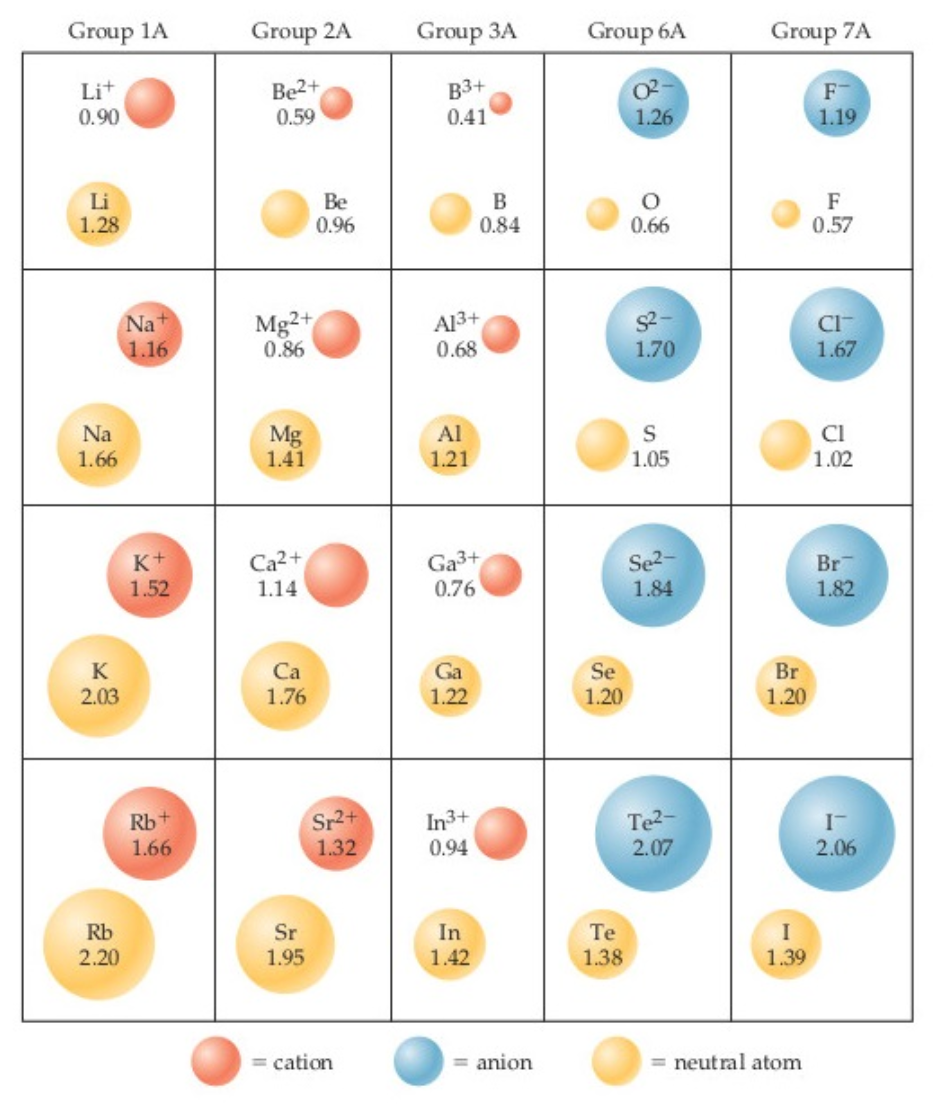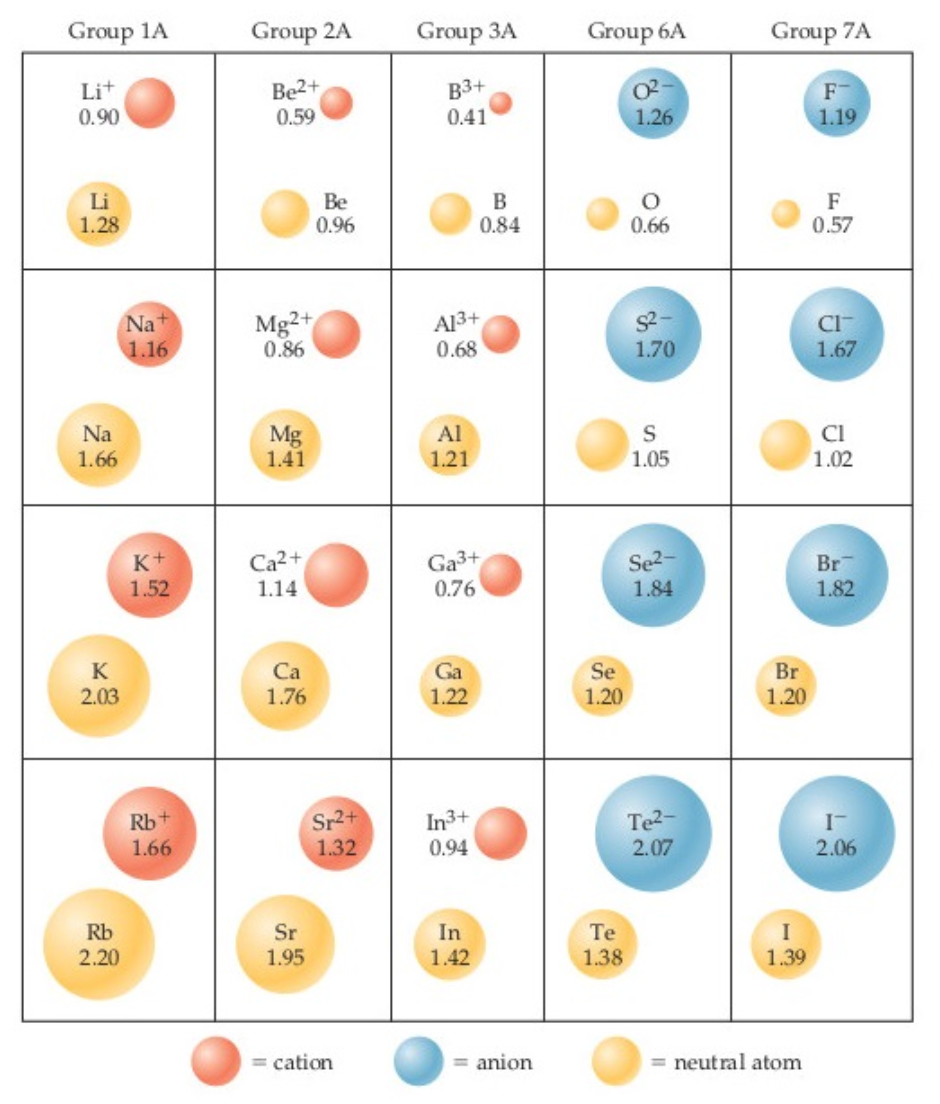# Problem: In the ionic compounds LiF, NaCl, KBr, and RbI, the measured cation-anion distances are 2.01 Å (Li - F), 2.82 Å (Na - Cl), 3.30 Å (K - Br), and 3.67 Å (Rb - I), respectively. Calculate the difference between the experimentally measured ion–ion distances and the ones predicted from the figure.

🤓 Based on our data, we think this question is relevant for Professor Dixon's class at UCF.

###### FREE Expert Solution

We are asked to  calculate the difference between the experimentally measured ion–ion distances and the ones predicted from the figure given:###### Problem Details
In the ionic compounds LiF, NaCl, KBr, and RbI, the measured cation-anion distances are 2.01 Å (Li - F), 2.82 Å (Na - Cl), 3.30 Å (K - Br), and 3.67 Å (Rb - I), respectively. Calculate the difference between the experimentally measured ion–ion distances and the ones predicted from the figure.What scientific concept do you need to know in order to solve this problem?

Our tutors have indicated that to solve this problem you will need to apply the Periodic Trends: Ionic Radius concept. You can view video lessons to learn Periodic Trends: Ionic Radius. Or if you need more Periodic Trends: Ionic Radius practice, you can also practice Periodic Trends: Ionic Radius practice problems.

What is the difficulty of this problem?

Our tutors rated the difficulty ofIn the ionic compounds LiF, NaCl, KBr, and RbI, the measured...as medium difficulty.

How long does this problem take to solve?

Our expert Chemistry tutor, Dasha took 6 minutes and 3 seconds to solve this problem. You can follow their steps in the video explanation above.

What professor is this problem relevant for?

Based on our data, we think this problem is relevant for Professor Dixon's class at UCF.